Scattering and tunnelling

Start this free course now. Just create an account and sign in. Enrol and complete the course for a free statement of participation or digital badge if available.

Free course

# 2.2 Wave packets and scattering in one dimension

Figure 6 shows the scattering of a wave packet, incident from the left, on a target represented by a potential energy function of the form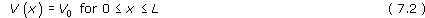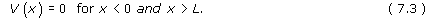Potential energy functions of this type are called finite square barriers. They are simple idealisations of the more general kind of finite barrier shown in Figure 4a. The de Broglie waves that make up the wave packet extend over a range of energy and momentum values. In the case illustrated in Figure 6, the expectation value of the energy, 〈E〉, has a value E0 that is greater than the height of the potential energy barrier V0. The classical analogue of the process illustrated in Figure 6 would be a particle of energy E0 scattering from a repulsive target. The unrealistically steep sides of the potential energy function imply that the encounter is sudden and impulsive – not like the encounter between two negatively charged particles, for instance; there is no gradual slope for the incident particle to ‘climb’, nor for it to descend after the interaction. Still, in the classical case, the fact that E0 is greater than V0, implies that the incident particle has enough energy to overcome the resistance offered by the target, and is certain to emerge on the far side of it.

The quantum analysis tells a different story. Based on numerical solutions of Schrödinger's equation, the computer-generated results in Figure 6 show successive snapshots of |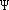|2, each of which represents the probability density of the particle at a particular instant. Examining the sequence of pictures it is easy to visualise the probability as a sort of fluid that flows from one part of the x-axis to another. Initially, as the wave packet approaches the barrier, the probability is concentrated in a single ‘blob’, flowing from left to right. Then, as the wave packet encounters the barrier, something odd starts to happen; the probability distribution develops closely spaced peaks. These peaks are a consequence of reflection – part of the quantum wave packet passes through the barrier, but another part is reflected; the reflected part interferes with the part still advancing from the left and results in the spiky graph of ||2. Eventually, however, the interference subsides and what remains are two distinct ‘blobs’ of probability density: one returning to the left, the other progressing to the right.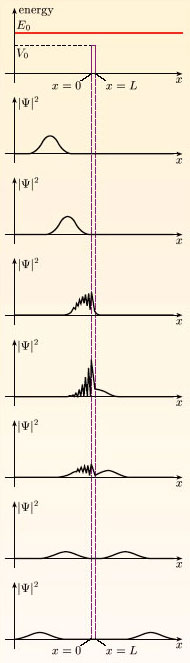Figure 6 The scattering of a wave packet with 〈E〉 = E0 by a finite square barrier of height V0 when E0 > V0. The probability density |Ψ|2 is shown in a sequence of snapshots with time increasing from top to bottom. The barrier has been made narrow in this example but a greater width could have been chosen

It's important to realise that the splitting of the wave packet illustrated in Figure 6 does not represent a splitting of the particle described by the wave packet. The normalisation of the wave packet is preserved throughout the scattering process (the area under each of the graphs in Figure 6 is equal to 1); there is only ever one particle being scattered. The splitting of the wave packet simply indicates that, following the scattering, there are two distinct regions in which the particle might be found. In contrast to the certainty of transmission in the classical case, the quantum calculation predicts some probability of transmission, but also some probability of reflection. Indeterminacy is, of course, a characteristic feature of quantum mechanics.

## Exercise 1

Simply judging by eye, what are the respective probabilities of reflection and transmission as the final outcome of the scattering process shown in Figure 6?

The two bumps in the probability density have roughly equal areas. This indicates that the probability of transmission is roughly equal to the probability of reflection. Since the sum of these two probabilities must be equal to 1, the probability of each outcome is about 0.5.

The probability that a given incident particle is reflected is called the reflection coefficient, R, while the probability that it is transmitted is called the transmission coefficient, T. Since one or other of these outcomes must occur, we always have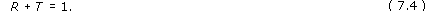The values of R and T that apply in any particular case of one-dimensional scattering can be worked out by examining the solution of the relevant Schrödinger equation that applies long after the incident particle has encountered the target. For properly normalised wave packets, the values of R and T are found by measuring the areas under the graph of ||2 that are located to the left and to the right of the target at that time.

Reflection and transmission coefficients can also describe scattering from an attractive target. The idealised case of a wave packet with 〈E〉 = E0 > 0 encountering a finite square well of depth V0 and width L is shown in Figure 7. Again, the wave packet is incident from the left, and the wave packet is partly reflected, giving rise to interference effects. Eventually, however, the interference abates, leaving two distinct parts of the wave packet. The areas of these two parts determine the reflection and transmission coefficients.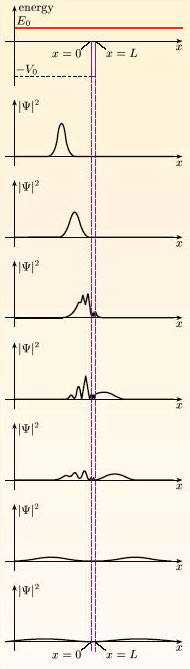Figure 7 The scattering of a wave packet with 〈E〉 = E0 by a finite square well of depth V0 when E0 > 0. The probability density |Ψ|2 is shown in a sequence of snapshots with time increasing from top to bottom. The well has been made narrow in this example but a greater width could have been chosen

Of course, wave mechanics is probabilistic, so although reflection and transmission coefficients can be calculated in any given situation, it is never possible to predict what will happen to any individual particle (unless R or T happen to be equal to zero in the situation considered). Indeed, the detection of the scattered particle, after the scattering has taken place, is exactly the kind of measurement that brings about the collapse of the wave function – a sudden, abrupt and unpredictable change that is not described by Schrödinger's equation. After such a collapse has occurred, the particle is localised on one side of the barrier or the other.

SM358_1